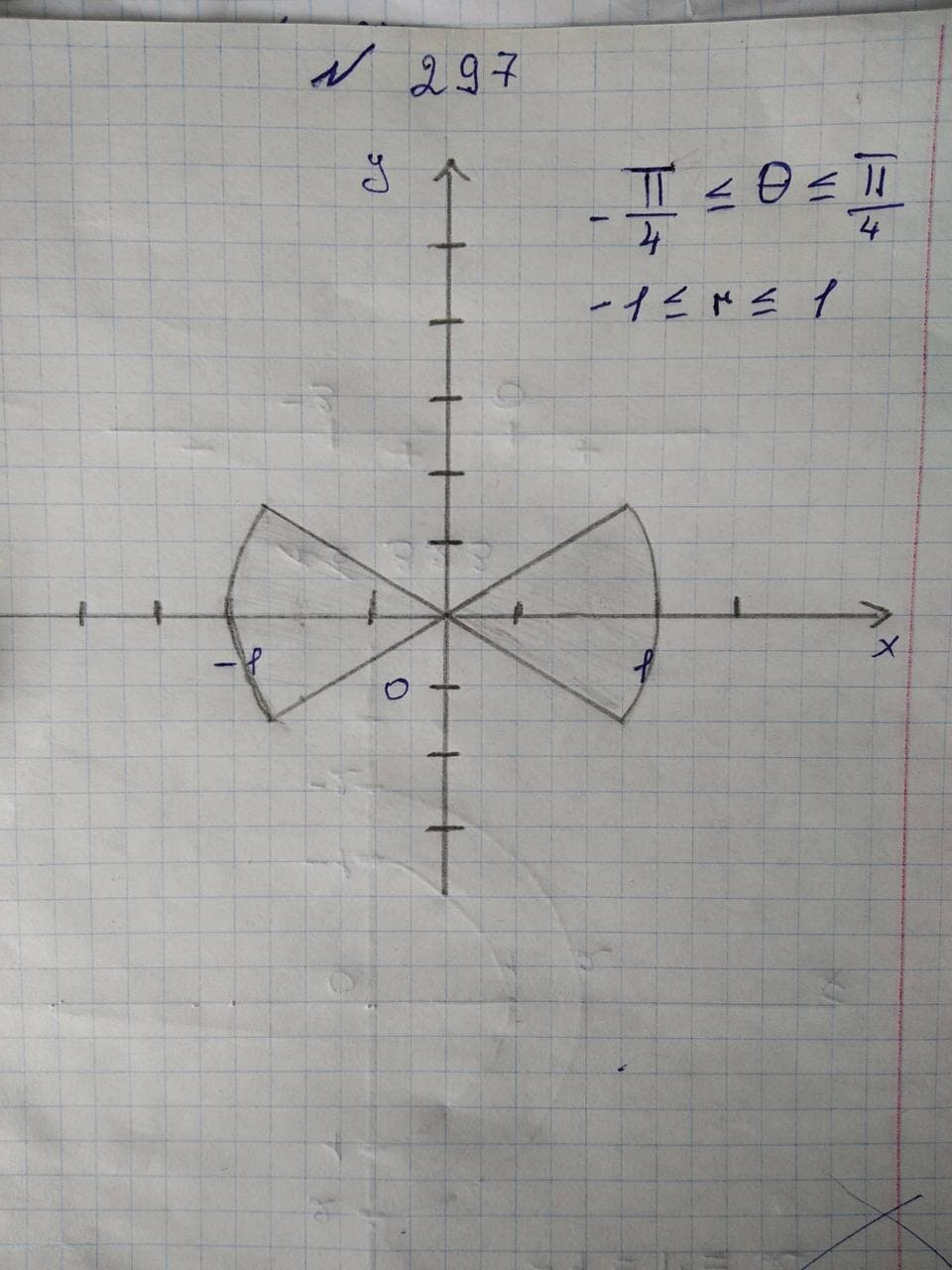Graph the sets of points whose polar coordinates satisfy theNompsypeFeplk 2021-11-19 Answered
Graph the sets of points whose polar coordinates satisfy the given polar equation.
The given polar equation is written as follows:
$$\displaystyle-{\frac{{\pi}}{{{4}}}}\le\theta\le{\frac{{\pi}}{{{4}}}},-{1}\le{r}\le{1}$$

• Questions are typically answered in as fast as 30 minutes

Solve your problem for the price of one coffee

• Math expert for every subject
• Pay only if we can solve itZachary Pickett

Consider the polar coordinates as $$\displaystyle{P}{\left({r},\theta\right)}.$$
Here, r is the directed distance from origin O to point Pand $$\theta$$ is the directed angle from initial ray to OP.
From the given equation, the value of $$\theta$$ varies from $$\displaystyle-{\frac{{\pi}}{{{2}}}}$$ to $$\displaystyle{\frac{{\pi}}{{{2}}}}$$ and r varies from 1 to 2.
Polar point traces a circle when r is fixed at constant value and it traces a straight line when $$\theta$$ is fixed at constant value.
From the analysis, draw the sets of points whose polar coordinates satisfy the given polar equation as shown in
Figure 1.